# Shading Toolbox #5 – Convolution Matrices

In this post we will talk about Filter Kernels, or Convolution Matrices. Filtering allows us to post process images and apply effects like Gaussian Blur, Sharpening or Edge Detection.

Hello readers! I mentioned a while back that we will talk about Convolution Matrices otherwise known as Filter Kernels.

Luckily, there isn’t all that much to talk about, but it would be a shame if a basic topic like that got lost somewhere inside a bigger post.

Let’s begin.

## The Basics

Convolution Matrices are usually small matrices, say 3×3 to 7×7, used to achieve visual filtering of an image. This can range from a Gaussian Blur filter to even an Emboss effect, yes, the one that was so hip in the early 2000’s flood of message board signature images and custom icons of pirated games. (Don’t ask me how I know this, a friend told me)

This filtering is achieved by convolution of an image upon itself, meaning, we fold the pixels around the pixel that we currently render onto that pixel. In easier words, averaging the color value of one pixel with it’s neighbors, but not all neighbors are equal in their influence on the average.

In any given convolution matrix, the middle value represents the weight of the pixel we currently look at. The other values inside the matrix represent the weight of the pixels around it. For each pixel we render, we apply said convolution matrix by adding all the pixels inside the kernels range of influence with their respective weight.

Let’s say we have a 3×3 matrix filled with the value 1. This would mean, that the color value of the pixel we will render is equal to the sum of our current pixel and the ones around it. (Which would not look very good.) However, if we multiply this matrix by 1/9, every pixel inside the kernel will only make up for 1/9 of the color. We effectively average the color of the pixel with it’s neighbors and the result is a „Box Blur“.

## Common Filter Kernels

So applying the principle above, here are some common convolution matrices, or filter kernels if you will in their basic 3×3 form. Some of them, like the Box Blur can easily be scaled up to 7×7 by simply repeating the pattern to get a better looking blur.

 Effect Matrix Identity (No Convolution / Effect)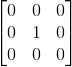Box Blur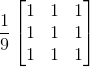Gaussian Blur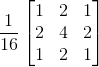Edge Detection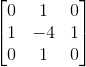Sharpen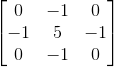Emboss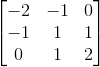Writing a general purpose filtering shader is pretty straight forward. Here is a fragment function and the needed declarations in Cg.

```// Main Texture
sampler2D _MainTex;
// texel size so we know the uv-size of one fragment
float4 _MainTex_TexelSize;

// Convolution Matrix
int m00, m01, m02,
m10, m11, m12,
m20, m21, m22;
// matrix multiplier
float c;

fixed4 frag (v2f i) : SV_Target
{
// Get the Pixels inside the Kernel, MainTex_TexelSize.x = the uv step needed to to one fragment on the x axis.
// If you don't have the TexelSize you can calculate it by 1/RenderingSize of the surface
// In post processing that would be 1/ScreenWidth for the x texel size and 1/ScreenHeight for the y texel size
fixed4 c00 = tex2D(_MainTex, i.uv + float2(-_MainTex_TexelSize.x, _MainTex_TexelSize.y));
fixed4 c01 = tex2D(_MainTex, i.uv + float2(0					, _MainTex_TexelSize.y));
fixed4 c02 = tex2D(_MainTex, i.uv + float2( _MainTex_TexelSize.x, _MainTex_TexelSize.y));

fixed4 c10 = tex2D(_MainTex, i.uv + float2(-_MainTex_TexelSize.x, 0));
fixed4 c11 = tex2D(_MainTex, i.uv + float2(0					, 0));
fixed4 c12 = tex2D(_MainTex, i.uv + float2( _MainTex_TexelSize.x, 0));

fixed4 c20 = tex2D(_MainTex, i.uv + float2(-_MainTex_TexelSize.x, -_MainTex_TexelSize.y));
fixed4 c21 = tex2D(_MainTex, i.uv + float2(0					, -_MainTex_TexelSize.y));
fixed4 c22 = tex2D(_MainTex, i.uv + float2( _MainTex_TexelSize.x, -_MainTex_TexelSize.y));

// compute the final color
return (
c00 * m00 + c01 * m01 + c02 * m02 +
c10 * m10 + c11 * m11 + c12 * m12 +
c20 * m20 + c21 * m21 + c22 * m22
) * c;

}```

Of course this is not necessarily the fastest way (performance wise) of achieving those effects, mind you, just a very convenient one where you can experiment with different kernel types.